# WELCOME BACK Math Club Session 2 Adrianna Hunt

• Slides: 15WELCOME (BACK)! Math Club Session 2 Adrianna Hunt and Alicia CharbonneauMission, vision, and core beliefs Edit as needed and sign the bottom pleaseThree Act Task: “Donut Delight” (This and MANY more can be found on the districts' math website under "Three Act Task" & are sorted by grade level/standard) : ) https: //tapintoteenminds. com/3 act-math/donut-delight/Twist & Talk What do we know about donuts? : ) (Think number-wise not just taste ha-ha)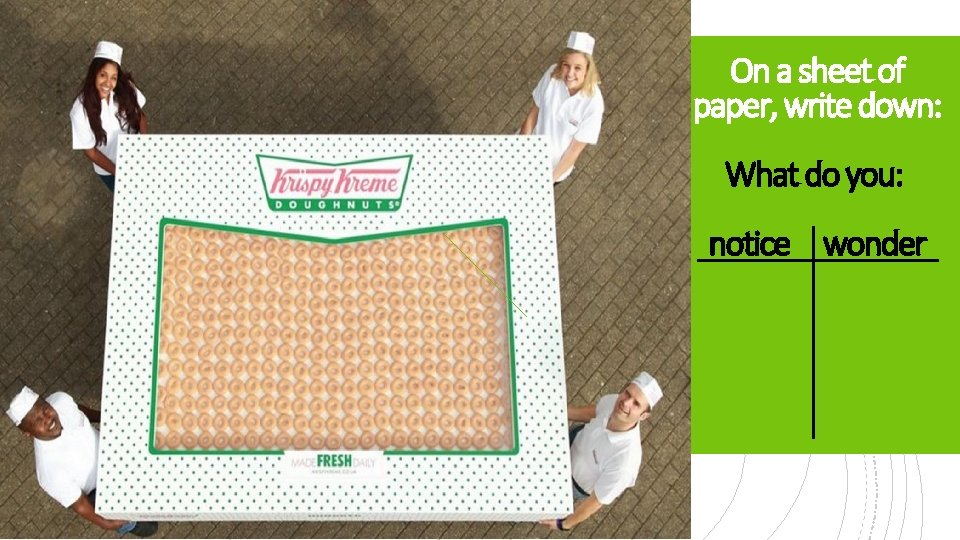On a sheet of paper, write down: What do you: notice wonder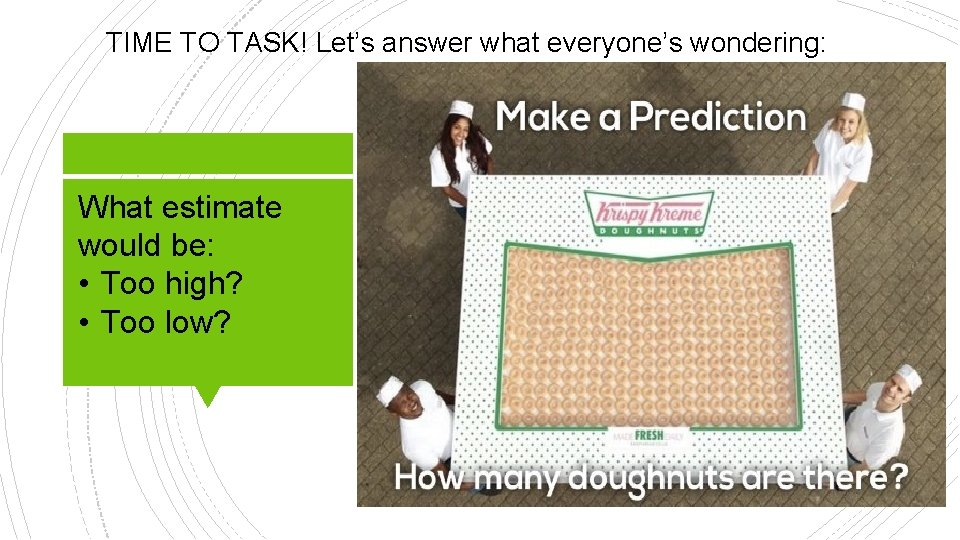TIME TO TASK! Let’s answer what everyone’s wondering: What estimate would be: • Too high? • Too low?Dough-NOT just guess, let’s be exact! What information do you need to help you find the exact number of donuts in this box?600 donuts!!!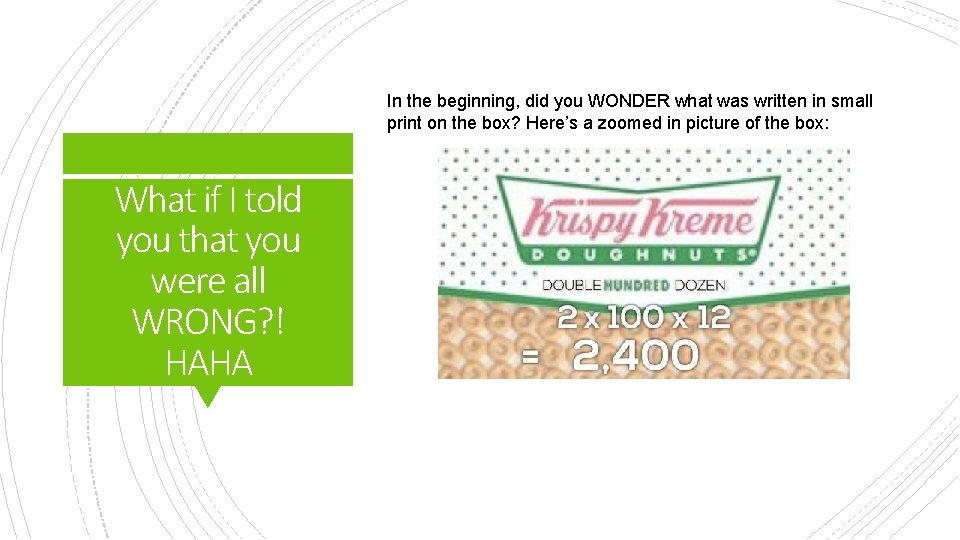In the beginning, did you WONDER what was written in small print on the box? Here’s a zoomed in picture of the box: What if I told you that you were all WRONG? ! HAHAHere’s an alternative to ENRICH their thinking and challenge them! Pull & Plan: Reread with your partner/table. PULL out what information you found valuable to our task and make a PLAN for how you intend to use it.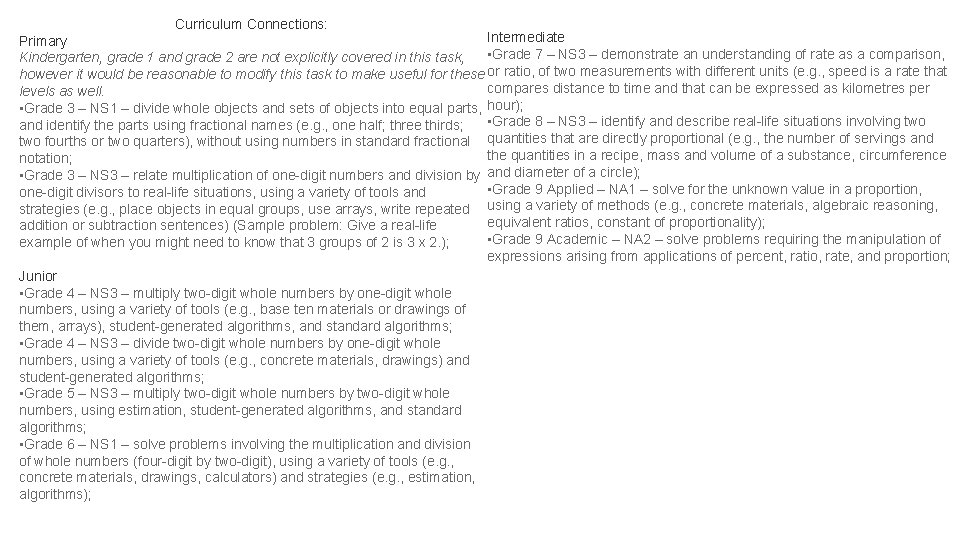Curriculum Connections: Intermediate Primary • Grade 7 – NS 3 – demonstrate an understanding of rate as a comparison, Kindergarten, grade 1 and grade 2 are not explicitly covered in this task, however it would be reasonable to modify this task to make useful for these or ratio, of two measurements with different units (e. g. , speed is a rate that compares distance to time and that can be expressed as kilometres per levels as well. • Grade 3 – NS 1 – divide whole objects and sets of objects into equal parts, hour); and identify the parts using fractional names (e. g. , one half; three thirds; • Grade 8 – NS 3 – identify and describe real-life situations involving two fourths or two quarters), without using numbers in standard fractional quantities that are directly proportional (e. g. , the number of servings and the quantities in a recipe, mass and volume of a substance, circumference notation; • Grade 3 – NS 3 – relate multiplication of one-digit numbers and division by and diameter of a circle); • Grade 9 Applied – NA 1 – solve for the unknown value in a proportion, one-digit divisors to real-life situations, using a variety of tools and strategies (e. g. , place objects in equal groups, use arrays, write repeated using a variety of methods (e. g. , concrete materials, algebraic reasoning, equivalent ratios, constant of proportionality); addition or subtraction sentences) (Sample problem: Give a real-life • Grade 9 Academic – NA 2 – solve problems requiring the manipulation of example of when you might need to know that 3 groups of 2 is 3 x 2. ); expressions arising from applications of percent, ratio, rate, and proportion; Junior • Grade 4 – NS 3 – multiply two-digit whole numbers by one-digit whole numbers, using a variety of tools (e. g. , base ten materials or drawings of them, arrays), student-generated algorithms, and standard algorithms; • Grade 4 – NS 3 – divide two-digit whole numbers by one-digit whole numbers, using a variety of tools (e. g. , concrete materials, drawings) and student-generated algorithms; • Grade 5 – NS 3 – multiply two-digit whole numbers by two-digit whole numbers, using estimation, student-generated algorithms, and standard algorithms; • Grade 6 – NS 1 – solve problems involving the multiplication and division of whole numbers (four-digit by two-digit), using a variety of tools (e. g. , concrete materials, drawings, calculators) and strategies (e. g. , estimation, algorithms);SEQUELS!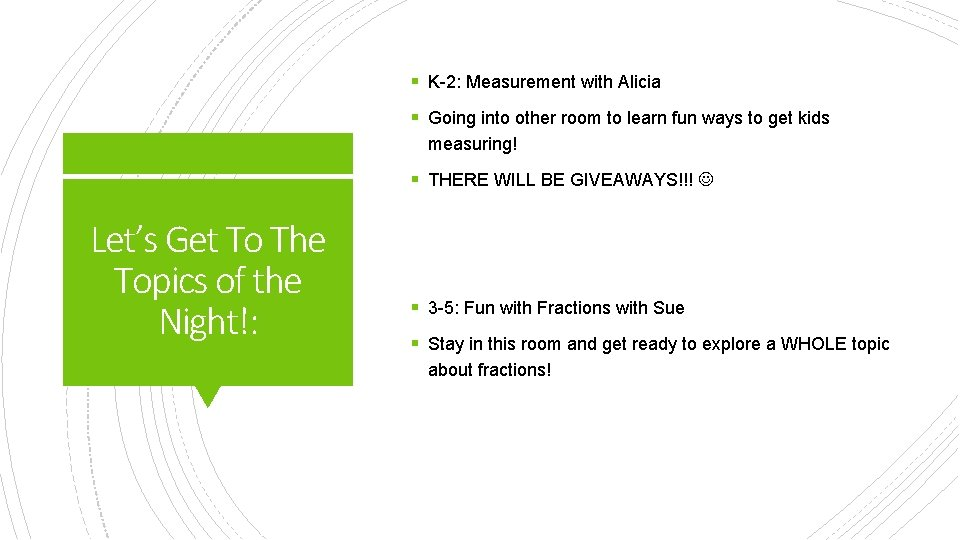§ K-2: Measurement with Alicia § Going into other room to learn fun ways to get kids measuring! § THERE WILL BE GIVEAWAYS!!! Let’s Get To The Topics of the Night!: § 3 -5: Fun with Fractions with Sue § Stay in this room and get ready to explore a WHOLE topic about fractions!Exit Ticket http: //bit. ly/Mathexit